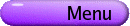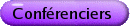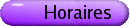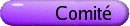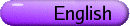Analyse matricielle
Org: Man-Duen Choi (Toronto) et Douglas Farenick (Regina)
[PDF]

TSUYOSHI ANDO, Hokkaido University, Sapporo, Japan
Indefinite Contractions
[PDF]

Given a matrix A, define the triple (p-(A),p0(A), p+(A)) as its inertia with respect to the unit circle where p-(A) (resp. p+(A)) is the number of eigenvalues of A inside (resp. outside) the unit disc while p0(A) is the number of eigenvalues on the unit circle.

An invertible Hermitian matrix H gives rise to an (indefinite) inner product. A matrix A is called an H-(strict) contraction (or H-contractive) if H > A* HA. The most interesing case is that H is an involution, H2 = I. We use J for H in such a case.

It is well known that a matrix A is H-contractive for suitable H if and only if p0(A) = 0. Though H is not determined uniquely by A (even up to positive scalar multiple), p-(A) must coincide with the number of positive eigenvalues of H.

If A is J-contractive, so is A* and hence A*A. Therefore A and its modulus |A| º (A*A)1/2 have the same inertia. But this property does not seem sufficient to guarantee J-contractivity of A for a suitable involution J. Other necessary conditions are presented.

If H-contractivity of a matrix A always guarantees that of its adjoint A* then H is necessarily a scalar multiple of an involution.

A characterization is given for a set of matrices coincides with the set of H-contractions for (unknown) H.

PAUL BINDING, University of Calgary
A generalized eigenvalue problem in the max-algebra
[PDF]

We consider the generalized eigenvalue problem

 AÄx = lBÄx,     x ³ 0, x ¹ 0,
where A and B are (entrywise) nonnegative n×n matrices, and the "max" product Ä satisfies
 (AÄx)i : = nmax m=1 aim xm.

The case B=I has been studied by several authors, and for irreducible (e.g., positive) A there is exactly one eigenvalue l in the above "max" sense.

The generalized problem is different, and for example neither existence nor uniqueness of eigenvalues is guaranteed, even for 2×2 positive matrices A and B. This case can be analysed by graphical methods, but for general n, degree theory turns out to be a more useful tool.

WAI-SHUN CHEUNG, The University of Hong Kong, Hong Kong
A matrix approach to the study of generalised derivatives of polynomials
[PDF]

Consider a polynomial p and a generalised derivative q of p. We construct two matrices whose characteristic polynomials are p and q respectively, and use the properties of the two matrices to study the relations between the zeros of p and the zeros of q. This approach is a generalization of the concept of differentiators recently revived by R. Pereira.

MAN-DUEN CHOI, University of Toronto, Department of Mathematics, Toronto, Ontario M5S 2E4
Completely Positive Linear Maps
[PDF]

This is a survey talk on the structure of completely positive linear maps, which serve as the natural morphisms in the category of matrix algebras (or operator algebras). It has been well known (for more than 30 years in electrical circuit theory), that completely positive linear maps, rather than positive linear maps, are useful connections for n-port networks. Incredibly, many new features of quantum operations (in recent quantum computing theory) turn out to be old and new structure problems of completely positive linear maps.

SHAUN FALLAT, Department of Mathematics & Statistics, University of Regina, Regina, SK S4S 0A2
Compressions of totally positive matrices
[PDF]

A matrix is called totally positive if all of its minors are positive. If a matrix A is partitioned as A = (Aij), i,j = 1,2,...,k, in which each block Aij is n ×n, then the k ×k compressed matrix is given by (detAij). It is well-known that if A is positive semidefinite, then the compressed matrix is also positive semidefinite and that the determinant of the compressed matrix is larger than detA. For a totally positive matrix A, we show that the compressed matrix is also totally positive and we verify that the determinant of the compressed matrix exceeds detA when k=2,3. An extension that allows for overlapping blocks is also presented when k=2,3. For k ³ 4 we verify, by example, that the k ×k compressed matrix of a totally positive matrix need not be totally positive.

DOUG FARENICK, University of Regina
Pure matrix states on operator systems
[PDF]

An operator system is a complex matricially ordered vector space that is completely order isomorphic to a unital selfadjoint subspace of a unital C*-algebra. A matrix state on an operator system V is a unital completely positive linear map of V into a full matrix algebra. Pure matrix states will be discussed, and a new and somewhat simplified proof of a Krein-Milman-type theorem of Webster and Winkler will be mentioned. If V is 3-dimensional, then the matrix state space of V is matrix-affinely homeomorphic to the matricial range of some Hilbert space operator. With the aid of this representation, pure matrix states on 3-dimensional operator systems are examined-and in some cases completely determined.

PETER GIBSON, York University, 4700 Keele Street, Toronto, ON M3J 1P3
[PDF]

We construct a family F of probability distributions on the real line for which iterated Gaussian quadrature, where the number of nodes is approximately doubled at each iteration, is computationally more efficient than usual. For each da in F, the 2n+1-point Gauss rule re-uses all the nodes of the n-point Gauss rule, the 2(2n+1)+1-point rule re-uses the nodes of the 2n+1-point rule, and so on indefinitely. We show that it is possible to construct a distribution of this type for an essentially arbitrary sequence of nodes. This implies, for example, the existence of a distribution supported on [-1,1] whose n-point Gauss rules have evenly spaced nodes, and (equivalently) whose orthogonal polynomials of degree n have evenly spaced zeros, for an unbounded sequence of indices n.

In addition we give an explicit construction for the subclass O F of F for which iteration of Gaussian quadrature re-uses, not only the nodes, but also the weights at each step. The classical distribution Ö{1-x2dx is derived as a particular example.

Inverse Positivity of Perturbed M-Matrices
[PDF]

A well known property of an M-matrix A is that the inverse is element-wise nonnegative, which we write as A-1 ³ 0. In this paper we consider diagonal perturbations of M-matrices and obtain bounds on the perturbations so that the nonnegative inverse persists.

STEVE KIRKLAND, University of Regina, Regina, Saskatchewan
Conditioning of the Entries in Google's PageRank Vector
[PDF]

The internet search engine Google approaches the problem of ranking web pages by computing an estimate of the Perron vector of a certain non-negative matrix associated with the world wide web. That Perron vector, known as the PageRank vector, is then used to rank the importance of the corresponding web pages. In this talk, we discuss the conditioning of the entries in the PageRank vector, and our results in turn lead to error bounds for Google's computed estimate of that vector. Our techniques involve non-negative matrices, generalized inverses, and combinatorial considerations.

IVO KLEMES, McGill University, 805 Sherbrooke St. W. # 1005, Montreal, QC H3A 2K6
Alexandrov's inequality and some conjectures on Toeplitz matrices
[PDF]

We prove the inequality det(QQ*) £ det(RR*) where Q is a certain Toeplitz matrix associated with the Dirichlet kernel on the unit circle, and R is any matrix of the same size as Q whose entries have modulus ³ 1 on the support of the entries of Q, zero otherwise. This is a special case of a similar problem for all of the elementary symmetric functions; Sk(QQ*) £ Sk(RR*), in the case when R is Toeplitz. The general case is still open. The proof uses Alexandrov's inequality on the polarized determinant (also known as the "mixed discriminant"). There are some connections with totally unimodular matrices, counting of bases modulo p, and Bazin-Reisz-Picquet determinant identities.

DAVID KRIBS, University of Guelph and Institute for Quantum Computing
Passive Quantum Error Correction
[PDF]

In this talk, I will discuss some of my recent work on error correction problems in quantum computing. I'll focus on the fundamental passive technique, which involves "decoherence-free subspaces" and "noiseless subsystems". In this method, symmetries within the noise of quantum channels are used to encode initial states in sectors of the system Hilbert space that are immune to the errors of the channel. The underlying mathematics involves completely positive maps and operator algebras.

PETER LANCASTER, University of Calgary
The Boundary of the Pseudo-spectrum
[PDF]

This work began with the design of an algorithm (work with P. Psarrakos) to trace the boundary of the pseudo spectrum for matrix poynomials. Here, the objective is to describe the nature of the boundary with respect to diferentiability, multiple points, and perturbations.

This work is in collaboration with P. Psarrakos and L. Boulton.

CHI-KWONG LI, College of William and Mary, Mathematics Department, Williamsburg, VA 23185, USA
Spectrum preserving maps
[PDF]

We will discuss recent results on spectrum preserving maps on matrices and operators, and related problems.

MATJAZ OMLADIC, University of Ljubljana, Dept. of Math., Jadranska 19, SI-1000 Ljubljana, Slovenia
Semigroups of matrices satisfying commutator equations
[PDF]

Some recently obtained reducibility results for matrix semigroups satisfying certain polynomial relations such as commutator relations will be discussed. For example, we will consider semigroups such that there is a matrix (not necessarily a member of the semigroup) with the property that the commutator with every member of the semigroup is nilpotent.

Majorization Relations in the Geometry of Polynomials
[PDF]

Majorization (in the sense of Hardy-Littlewood-Polya and Marshall-Olkin) is an important concept in matrix analysis and the theory of inequalities. In this talk, we show how matrix analysis can be used to derive majorization relations between the zeros and the critical points of polynomials. We will examine both recent work in this area as well as applications to classical problems in the geometry of polynomials such as the spans of hyperbolic polynomials and Mahler measure inequalities.

HEYDAR RADJAVI, University of Waterloo, Waterloo, Ontario
An Approximate Version of Specht's Theorem
[PDF]

Specht's theorem states that two complex matrices are unitarily equivalent if and only if all traces of words in two noncommutative letters applied to the pairs (A,A*) and (B,B*) coincide. In joint work with Laurent Marcoux and Mitja Mastnak, we study an approximate version of this trace hypothesis and obtain conditions that allow us to extend this theorem to simultaneous similarity or unitary equivalence of families of operators.

PETER ROSENTHAL, University of Toronto, Toronto, ON
Equations such as AX + YB = Z in Matrices and Operators
[PDF]

Let A and B be square matrices of the same size. It is easily seen that the mapping that sends each pair (X,Y) of square matrices into the matrix AX + YB is onto if and only if at least one of A and B is invertible. The analogue is established for operators on Hilbert space, and partial results are obtained for more general operator equations in several variables.

This is joint work with Don Hadwin and Eric Nordgren.

PETER SEMRL, University of Ljubljana, Dept. of Math., Jadranska 19, SI-1000 Ljubljana
Maps on matrix spaces
[PDF]

Some recently obtained structural results for maps on matrix algebras (or their subsets) having certain algebraic or preserving properties will be presented. For example, we will consider maps on the set of idempotents preserving usual partial order or orthogonality. Connections with physics and geometry will be disscused.

MICHAEL TSATSOMEROS, Washington State University, Mathematics, Pullman, WA 99164-3113, USA
The Principal Minor Assignment Problem
[PDF]

We will consider the following inverse problem:

[PMAP] Find, if possible, an n×n matrix A having prescribed principal minors.
We will discuss conditions for the solvability of PMAP, an algorithm to compute a solution, and consequences in classical problems.